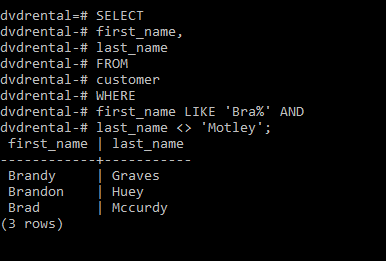Open In App
Related Articles
• Write an Interview Experience

# PostgreSQL – WHERE clause

The PostgreSQL WHERE clause is used to filter results returned by the SELECT statement.

Syntax: SELECT select_list FROM table_name WHERE condition;

Let’s analyze the above syntax:

• The WHERE clause appears right after the FROM clause of the SELECT statement
• The condition evaluates to true, false, or unknown. It can either be a Boolean expression or a combination of Boolean expressions where AND and OR operators are used.
• The WHERE clause can also be used with the UPDATE and DELETE statement to specify rows to be updated or deleted.

Below table provides us with the list of comparison operators valid in PostgreSQL:

OperatorDescription
=Equal
>Greater than
<Less than
>=Greater than or equal to
<=Less than or equal to
<> or =!Not equal
ANDLogical AND operator
ORLogical OR operator

For the sake of this article we will be using the sample DVD rental database, which is explained here and can be downloaded by clicking on this link.
Now, let’s look into some examples.

Example 1:
Using WHERE clause with the equal (=) operator. Here we will be using the equal operator in the “customer” table of our sample database.

```SELECT
last_name,
first_name
FROM
customer
WHERE
first_name = 'Kelly';```

Output: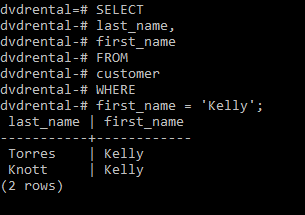Example 2:
Using the WHERE clause with the AND operator. Here we will be using the AND operator in the “customer” table of our sample database.

```SELECT
last_name,
first_name
FROM
customer
WHERE
first_name = 'Kelly'
AND last_name = 'Knott';```

Output: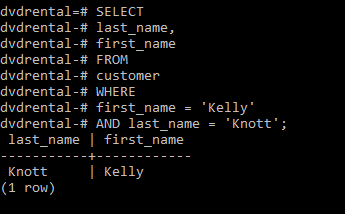Example 3:
Using the WHERE clause with the OR operator. Here we will be using the OR operator in the “customer” table of our sample database.

```SELECT
first_name,
last_name
FROM
customer
WHERE
last_name = 'Cooper' OR
first_name = 'Jo';```

Output: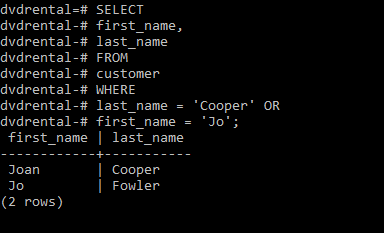Example 4:
Using the WHERE clause with the IN operator. The IN operator is used for string matching. Here we will be using the IN operator in the “customer” table of our sample database.

```SELECT
first_name,
last_name
FROM
customer
WHERE
first_name IN ('Kelly', 'Jo', ' Alexander');```

Output: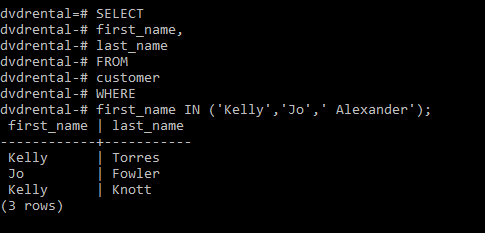Example 5:
Using the WHERE clause with the LIKE operator. The LIKE operator is used to find string matching a particular pattern. Here we will be using the LIKE operator in the “customer” table of our sample database.

```SELECT
first_name,
last_name
FROM
customer
WHERE
first_name LIKE 'Kath%';```

Output: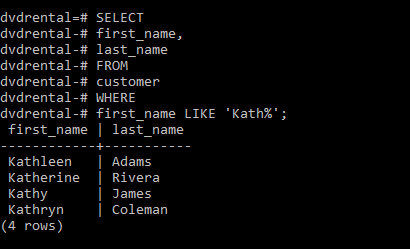Example 6:
Using the WHERE clause with the BETWEEN operator. The BETWEEN operator return if a value is in the mentioned range. Here we will be using the BETWEEN operator in the “customer” table of our sample database.

```SELECT
first_name,
LENGTH(first_name) name_length
FROM
customer
WHERE
first_name LIKE 'K%' AND
LENGTH(first_name) BETWEEN 3 AND 7
ORDER BY
name_length;```

Output: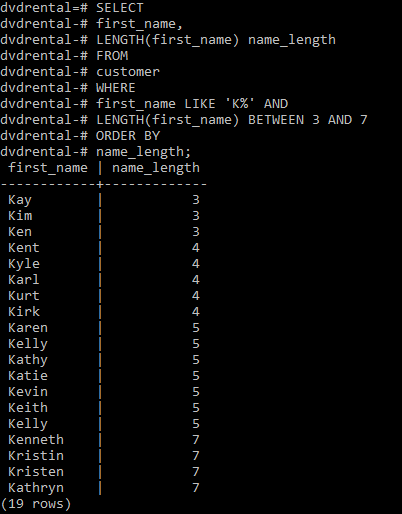Example 7:
Using the WHERE clause with the not equal operator (<>). Here we will be using the <> operator in the “customer” table of our sample database.

```SELECT
first_name,
last_name
FROM
customer
WHERE
first_name LIKE 'Bra%' AND
last_name <> 'Motley';```

Output: